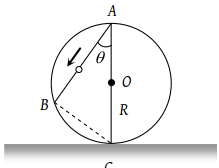A boat is moving with velocity of $3\stackrel{^}{i}+4\stackrel{^}{j}$ in river and water is moving with a velocity of $-3\stackrel{^}{i}-4\stackrel{^}{j}$ with respect to ground. Relative velocity of boat with respect to water is:

(1) $-6\stackrel{^}{i}-8\stackrel{^}{j}$

(2) $6\stackrel{^}{i}+8\stackrel{^}{j}$

(3) $8\stackrel{^}{j}$

(4) $6\stackrel{^}{i}$

Subtopic:  Relative Motion |
To view explanation, please take trial in the course below.
NEET 2022 - Target Batch - Aryan Raj Singh
To view explanation, please take trial in the course below.
NEET 2022 - Target Batch - Aryan Raj Singh

Difficulty Level:

A boat moves with a speed of 5 km/h relative to water in a river flowing with a speed of 3 km/h and having a width of 1 km. The minimum time taken around a round trip(returning to the initial point) is:

(1) 5 min

(2) 60 min

(3) 20 min

(4) 30 min

Subtopic:  Relative Motion |
To view explanation, please take trial in the course below.
NEET 2022 - Target Batch - Aryan Raj Singh
To view explanation, please take trial in the course below.
NEET 2022 - Target Batch - Aryan Raj Singh

Difficulty Level:

A river is flowing from W to E with a speed of 5 m/min. A man can swim in still water with a velocity 10 m/min. In which direction should the man swim so as to take the shortest possible path to go to the south.

(1) 30° with downstream

(2) 60° with downstream

(3) 120° with downstream

(4) South

Subtopic:  Relative Motion |
To view explanation, please take trial in the course below.
NEET 2022 - Target Batch - Aryan Raj Singh
To view explanation, please take trial in the course below.
NEET 2022 - Target Batch - Aryan Raj Singh

Difficulty Level:

A train is moving towards east and a car is along north, both with same speed. The observed direction of car to the passenger in the train is

(1) East-north direction

(2) West-north direction

(3) South-east direction

(4) None of these

Subtopic:  Relative Motion |
To view explanation, please take trial in the course below.
NEET 2022 - Target Batch - Aryan Raj Singh
To view explanation, please take trial in the course below.
NEET 2022 - Target Batch - Aryan Raj Singh

Difficulty Level:

A ball P is dropped vertically and another ball Q is thrown horizontally from the  same height and at the same time. If air resistance is neglected, then

(1) Ball P reaches the ground first

(2) Ball Q reaches the ground first

(3) Both reach the ground at the same time

(4) The respective masses of the two balls will decide the time

Subtopic:  Projectile Motion |
To view explanation, please take trial in the course below.
NEET 2022 - Target Batch - Aryan Raj Singh
To view explanation, please take trial in the course below.
NEET 2022 - Target Batch - Aryan Raj Singh

Difficulty Level:

A frictionless wire AB is fixed on a sphere of radius R. A very small spherical ball slips on this wire. The time taken by this ball to slip from A to B is(1) $\frac{2\sqrt{gR}}{g\mathrm{cos}\theta }$

(2) $2\sqrt{gR}.\frac{\mathrm{cos}\theta }{g}$

(3) $2\sqrt{\frac{R}{g}}$

(4) $\frac{gR}{\sqrt{g\mathrm{cos}\theta }}$

Subtopic:  Uniformly Accelerated Motion |
To view explanation, please take trial in the course below.
NEET 2022 - Target Batch - Aryan Raj Singh
To view explanation, please take trial in the course below.
NEET 2022 - Target Batch - Aryan Raj Singh

Difficulty Level:

A body is slipping from an inclined plane of height h and length l. If the angle of inclination is θ, the time taken by the body to come from the top to the bottom of this inclined plane is

(1) $\sqrt{\frac{2h}{g}}$

(2) $\sqrt{\frac{2l}{g}}$

(3) $\frac{1}{\mathrm{sin}\theta }\sqrt{\frac{2h}{g}}$

(4) $\mathrm{sin}\theta \sqrt{\frac{2h}{g}}$

Subtopic:  Acceleration |
To view explanation, please take trial in the course below.
NEET 2022 - Target Batch - Aryan Raj Singh
To view explanation, please take trial in the course below.
NEET 2022 - Target Batch - Aryan Raj Singh

Difficulty Level:

An aeroplane is moving with a velocity u. It drops a packet from a height h. The time t taken by the packet in reaching the ground will be

(1) $\sqrt{\left(\frac{2g}{h}\right)}$

(2) $\sqrt{\left(\frac{2u}{g}\right)}$

(3) $\sqrt{\left(\frac{h}{2g}\right)}$

(4) $\sqrt{\left(\frac{2h}{g}\right)}$

Subtopic:  Projectile Motion |
To view explanation, please take trial in the course below.
NEET 2022 - Target Batch - Aryan Raj Singh
To view explanation, please take trial in the course below.
NEET 2022 - Target Batch - Aryan Raj Singh

Difficulty Level:

An aeroplane is moving with horizontal velocity u at height h. The velocity of a packet dropped from it on the earth's surface will be (g is acceleration due to gravity)

(1) $\sqrt{{u}^{2}+2gh}$

(2) $\sqrt{2gh}$

(3) 2 gh

(4) $\sqrt{{u}^{2}-2gh}$

Subtopic:  Projectile Motion |
To view explanation, please take trial in the course below.
NEET 2022 - Target Batch - Aryan Raj Singh
To view explanation, please take trial in the course below.
NEET 2022 - Target Batch - Aryan Raj Singh

Difficulty Level:

A bullet is fired with a speed of $1000\text{\hspace{0.17em}\hspace{0.17em}}m/\mathrm{sec}$ in order to hit a target 100 m away. If $g=10\text{\hspace{0.17em}\hspace{0.17em}}m/{s}^{2}$, the gun should be aimed:

(1) Directly towards the target

(2) 5 cm above the target

(3) 10 cm above the target

(4) 15 cm above the target

Subtopic:  Projectile Motion |
To view explanation, please take trial in the course below.
NEET 2022 - Target Batch - Aryan Raj Singh
To view explanation, please take trial in the course below.
NEET 2022 - Target Batch - Aryan Raj Singh

Difficulty Level: